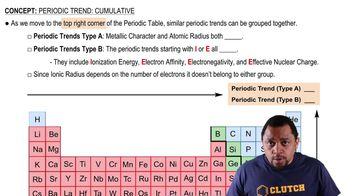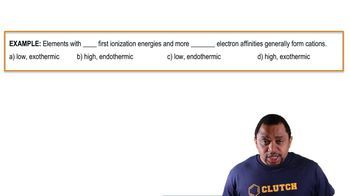Start typing, then use the up and down arrows to select an option from the list.
2:19 minutes
Problem 54
Textbook Question

# Consider the following equation: Al3+1g2 + e-¡Al2+1g2 Which of the following statements are true? (i) The energy change for this process is the second electron affinity of Al atom since Al2+1g2 is formed. (ii) The energy change for this process is the negative of the third ionization energy of the Al atom. (iii) The energy change for this process is the electron affinity of the Al2+ ion.Verified Solution
This video solution was recommended by our tutors as helpful for the problem above.
171views